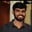Related Tags

matrix
c
communitycreator

# How to subtract two matrices in CBuchiredddypalli Koushik

### Problem overview

Read two matrices using the standard input (scanf) and subtract the two matrices to get the result. This result should be stored in the new matrix.

### Example

Let’s first take 2x2 matrices and try to subtract them.

Matrix 1:

[8  6]
[5  4]


Matrix 2:

 [1  5]
[2  1]


The resultant matrix looks like:

#Resultant Matrix : Matrix 1 - Matrix 2
[7  1]
[3  3]


### Algorithm

We can subtract only two square matrices. Subtraction of two matrices means that we must subtract the corresponding elements of the two matrices.

Note: Square matrices are the ones in which we have the exact dimensions. Both the matrices should be of the same dimension.

When we read the data, we need to use the loops. We also need the loops to access the corresponding elements of the matrices. After accessing the elements, we’ll subtract the elements and store the data in the resultant matrix.

The pseudo-code of the algorithm is as follows:

for(i=0 ; i<n ; i++)
{
for(j=0 ; j<n ; j++)
{
Resultant[i][j]= Matrix1[i][j]-Matrix2[i][j];
}
}


### Notations

In the above code:

• n: Represents the size of the matrix.

• Matrix1[n][n]: Represents the Matrix 1.

• Matrix2[n][n]: Represents the Matrix 2.

• Resultant[n][n]: Represents the subtraction of two matrices or the resultant matrix.

The algorithm is explained for 2x2 dimension matrices in the slides below:

Subtraction of Matrices
1 of 5

### Inputs for the code

To run the code below, first give the input value of n (size), followed by values of the two matrices. After giving all the inputs, we get the resultant matrix which is the subtraction of Matrix1 and Matrix2.

#include<stdio.h>
int main()
{
int i,j,n;
scanf("%d",&n);
printf("The value of n is : %d\n",n);
int Matrix1[n][n],Matrix2[n][n],Resultant[n][n];
printf("The 1st Matrix is :\n");
for(i=0;i<n;i++)
{
for(j=0;j<n;j++)
{
scanf("%d",&Matrix1[i][j]);
printf("%d ",Matrix1[i][j]);
}
printf("\n");
}
printf("The 2nd Matrix is :\n");
for(i=0;i<n;i++)
{
for(j=0;j<n;j++)
{
scanf("%d",&Matrix2[i][j]);
printf("%d ",Matrix2[i][j]);
}
printf("\n");
}

for(i=0;i<n;i++)
{
for(j=0;j<n;j++)
{
Resultant[i][j]=Matrix1[i][j]-Matrix2[i][j];

}

}
printf("The Resultant Matrix is :\n");
for(i=0;i<n;i++)
{
for(j=0;j<n;j++)
{

printf("%d ",Resultant[i][j]);
}
printf("\n");
}
}


Enter the input below

Code for the subtraction of matrices

RELATED TAGS

matrix
c
communitycreator

CONTRIBUTORBuchiredddypalli Koushik
RELATED COURSES

View all Courses

Keep Exploring

Learn in-demand tech skills in half the time